# Impossible figures in the real world

Many people thinks that impossible figures are really impossible and they cannot be represented in the real world. Nevertheless, we know that any draft is a projection of a three-dimensional object on a plane. Therefore, any figure drawn on a paper must have a corresponding figure (or figures) in three-dimensional space. Moreover, there are infinite number of three-dimensional objects which can be projected on a plane into the same figure. Impossible figures are not exception.

Of course, none of the impossible figures cannot be created in space by simple way. For example, you cannot take three wood blocks and join them as they constitute impossible triangle. But in plane projection of a three-dimensinal object some edges can be overlaped by other edges, and some curved edges can become straight lines.

Using this principle we can join three wood bars as it represented on fig. 1. This photo was made by mathematician from Holland and famous popularizer of Escher's artworks Bruno Ernst. In the foreground we see impossible triangle and in the background we see the same figure in the mirror. In the mirror we see the same figure from other point of view. And from other point of view we can see that figure of impossible triangle is not closed figure. So can see impossible triangle as impossible figure from one point of view only. If we shifted our view we see the gap between bars. The fact of impossible triangle looks impossible from one point of view only is inherent to all impossible figures.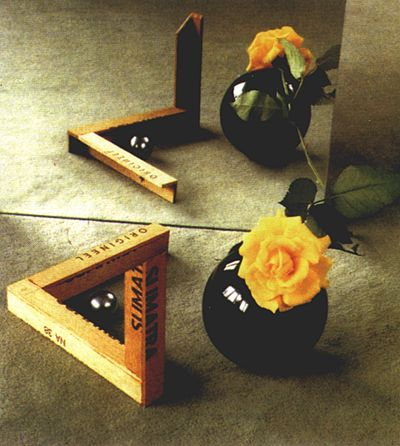Fig. 1. A photo of impossible triangle made by Bruno Ernst

As it was said before, there are infinite number of three-dimensinal objects corresponding to the same impossible figure. On fig. 2 you can see another implementation of impossible triangle. This photo was made by Belgian mathematician Mathieu Hamaekers. The left picture shows three-dimensional object from such angle as it looks as impossible triange. The last two photos shows the same object rotated by 45° and 90° correspondingly.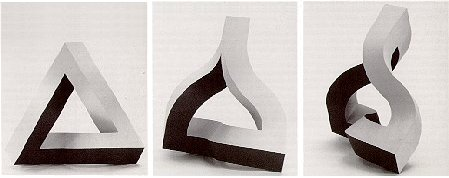Fig. 2. The impossible triangle created by Mathieu Hamaekers.

As you can see, there's no straight lines in this object. The object looks impossible from single point of view only where all curved edges became straight lines. Disregarding shadows the figure looks like impossible triangle.

Yet another way to construct impossible triangle was first suggested by russian artist and constructor Viacheslav Koleychuk, which was published in magazine "Tekhnicheskaya Estetika" (N9, 1974). In his version of triangle all edges were straight and surfaces distorted. Wooden example of this model you can see below.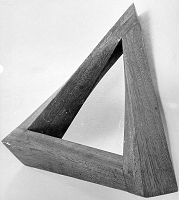Fig. 3. Wooden impossible triangle by Viacheslav Koleychuk

Later this kind of triangle was created by Elber Gershon a member of Computer Science faculty of Technion institute in Israel. He designed computer model and printed it on 3D-printer. You can see his two images of impossible triangle from two points of view below.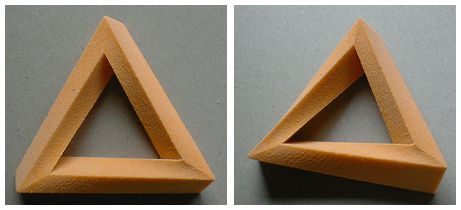Fig. 4. Impossible Triangle by Elber Gershon

It is significant, if we examine these objects from necessary point of view instead photos then we see that these objects are not impossible. Having stereoscopic vision we see objects from two points of view at one time. Our brain combines two different images from our eyes into one image and we see all tricks of these figures.

Is it true, that we cannot observe impossible figures in the real world? No, we can. If you hide one eye, you can observe an object from one point of view. Thus you can find necessary point of view where object will look impossible. And so, at exhibitions visitors forced to see impossible figures by one eye through small hole.

There's another way to see impossible figures. Using this way you can see impossible figures by both eyes. It's necessary to create huge object and look on it from long distance. In this case, you can observe figure by both eyes and perceive it as impossible because your eyes receives approximately identical images. In Perth (Australia) a sculpture of impossible triangle was created using this principle.

It's more difficult to create impossible trident in the real world. The distinctive feature of the impossible trident is contradiction between foreground and background. Some elements of the figure smoothly transfers from foreground to background and vice versa.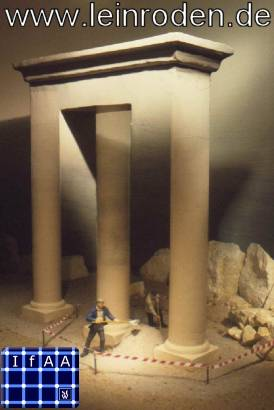Scientists of Eye Optics Institute in Aahen (Germany) created special construction which allows to see the scene from fig. 4 in reality. The construction divided into two parts by half-permeable mirror. There are three cylindrical columns and builder man figure in the front part which is illuminated at the bottom. There is half-permeable mirror behind three columns. Reflective layer on the front of the mirror prevents observer to see what is behind the mirror.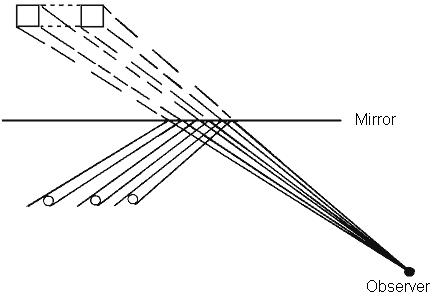Fig. 6. Half-permeable mirror

The image in the mirror changes when sources of light are turn on. Then two rectangular columns and transversal bar become visible through half-permeable layer. The back part of the scene is illuminated at the top.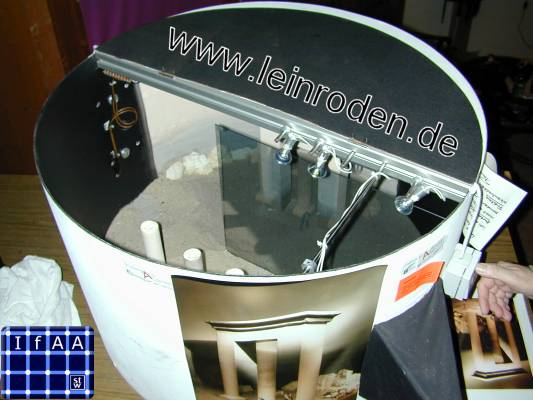Fig. 7. Photo of the construction, which represents impossible scene.

Observer have to look at mirror and see reflections of cylindrical columns but not columns itself. Front and back parts of the scene are situated in such way that outlines of columns coincided.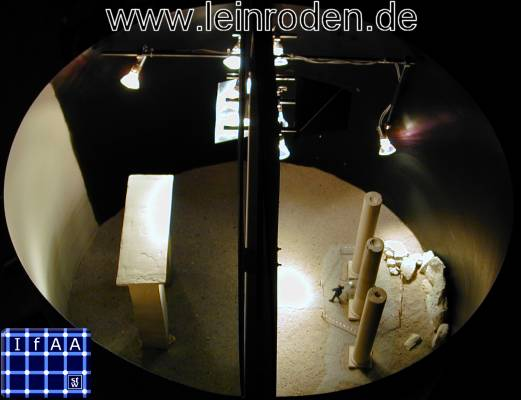Fig. 8. Photo of the construction, which represents impossible scene.